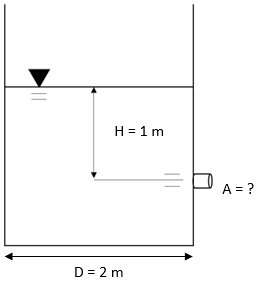## Drainage Time

The figure shown is a cross sectional view of a circular water tank with a 2 m diameter that discharges water freely into the atmosphere. If the time required to drain the water line from 1 m to 0.5 m is 1 minute, what is the output nozzle’s cross-sectional area in square cm? Assume no minor losses in discharge.Hint
The time required to drain a circular tank:
$$\Delta t=\frac{2(A_t/A_0)}{\sqrt{2g}}(h_{1}^{0.5}-h_{2}^{0.5})$$$where $$A_t$$ is the tank’s cross-sectional area, $$A_0$$ is the cross-sectional area of fluid flow, $$g$$ is the acceleration due to gravity, $$h_1$$ is the initial fluid line height, and $$h_2$$ is the final fluid line height. Hint 2 $$A_t=\frac{\pi D_{t}^{2}}{4}$$$
where $$D_t$$ is the circular tank’s diameter.
First, let’s find the tank’s cross-sectional area:
$$A_t=\frac{\pi D_{t}^{2}}{4}$$$where $$D_t$$ is the circular tank’s diameter. $$A_t=\frac{\pi (2m)^{2}}{4}=3.14\:m^2$$$
The time required to drain a circular tank:
$$\Delta t=\frac{2(A_t/A_0)}{\sqrt{2g}}(h_{1}^{0.5}-h_{2}^{0.5})$$$where $$A_t$$ is the tank’s cross-sectional area, $$A_0$$ is the cross-sectional area of fluid flow, $$g$$ is the acceleration due to gravity, $$h_1$$ is the initial fluid line height, and $$h_2$$ is the final fluid line height. $$1min\cdot \frac{60sec}{1min}=\frac{2(3.14m^2/A_0)}{\sqrt{2(9.8m/s^2)}}((1m)^{0.5}-(0.5m)^{0.5})$$$
Solving for the nozzle’s cross-sectional area:
$$60sec=\frac{2(3.14m^2/A_0)}{\sqrt{19.6m/s^2}}(1\sqrt{m}-0.707\sqrt{m})$$$$$60s=\frac{2(3.14m^2/A_0)}{4.43\frac{\sqrt{m}}{s}}(0.293\sqrt{m})$$$
$$\frac{60s(4.43s^{-1})}{(0.293)}=\frac{6.28m^2}{A_0}$$$$$A_0=\frac{6.28m^2}{907.17}=0.0069m^2=69\:cm^2$$$
$$69\:cm^2$$\$